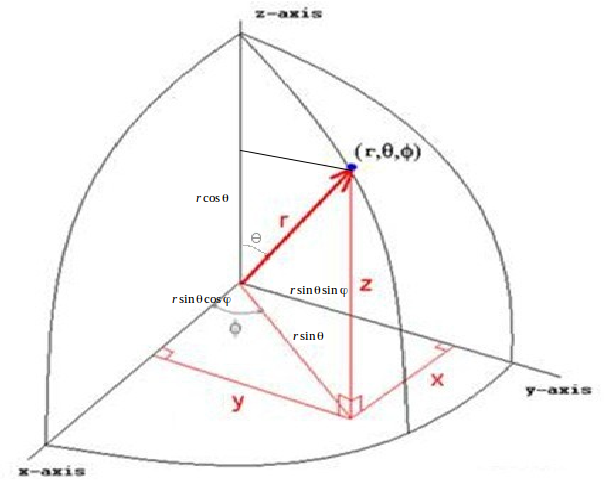## Spherical Coordinates

We need three number to define a point in three dimensional space, but ordinary Cartesian coordinates with mutually perpendicular axes is not always the best system to use. One alternative is the system of spherical coordinates. This is especially useful when problems have spherical symmetry or distance from a point. belowWe can write a point defined is spherical coordinates
$(r, \theta , \phi )$
is Cartesian coordinates.
$x= r sin \theta cos \phi$

$x= r sin \theta sin \phi$

$x= r cos \theta$